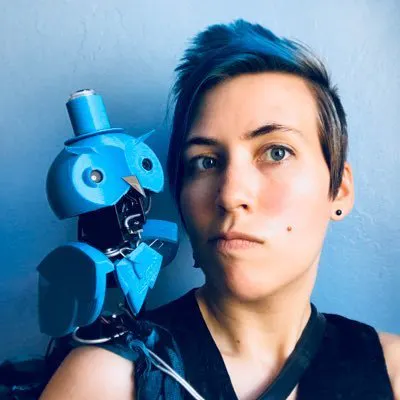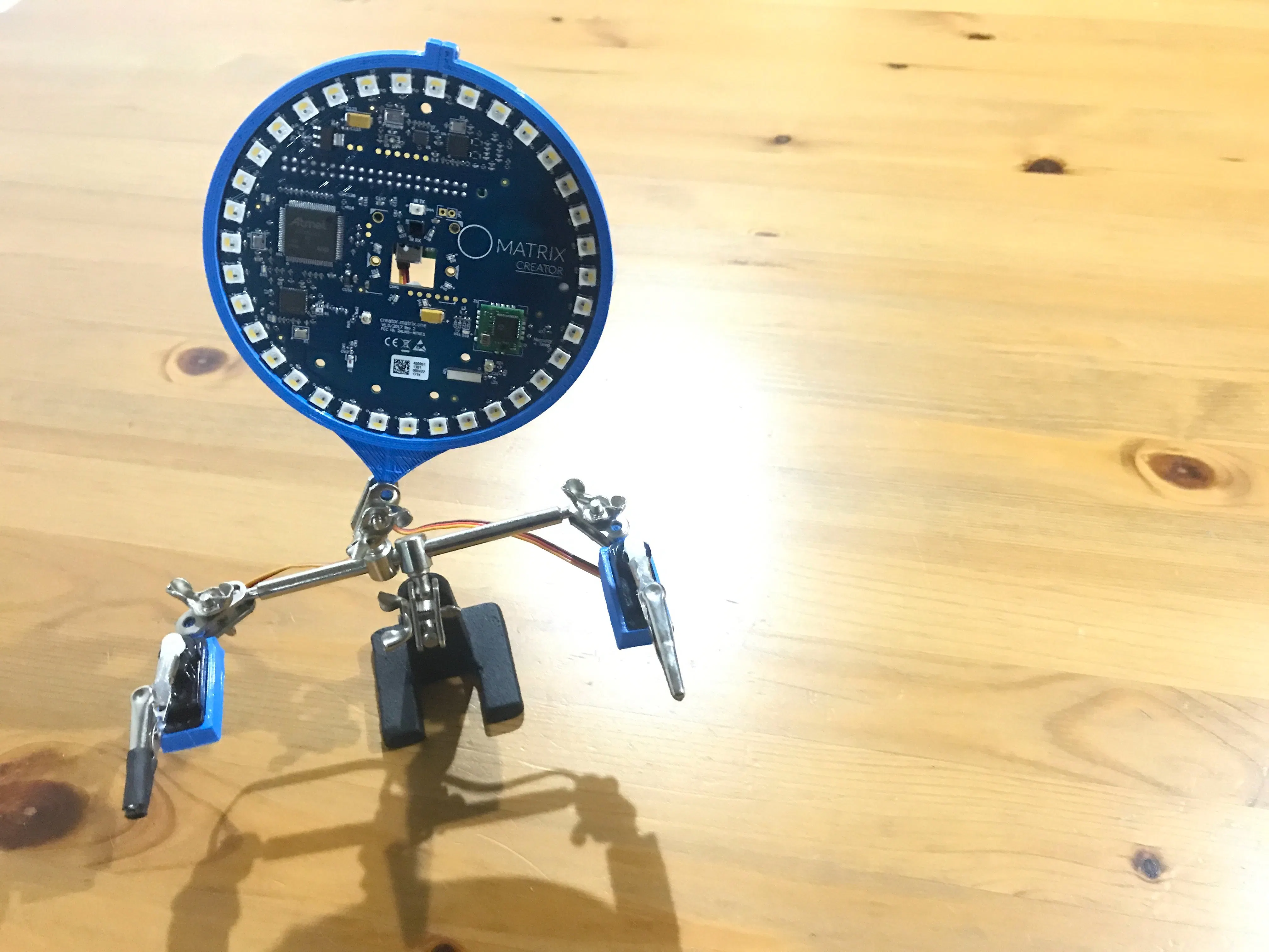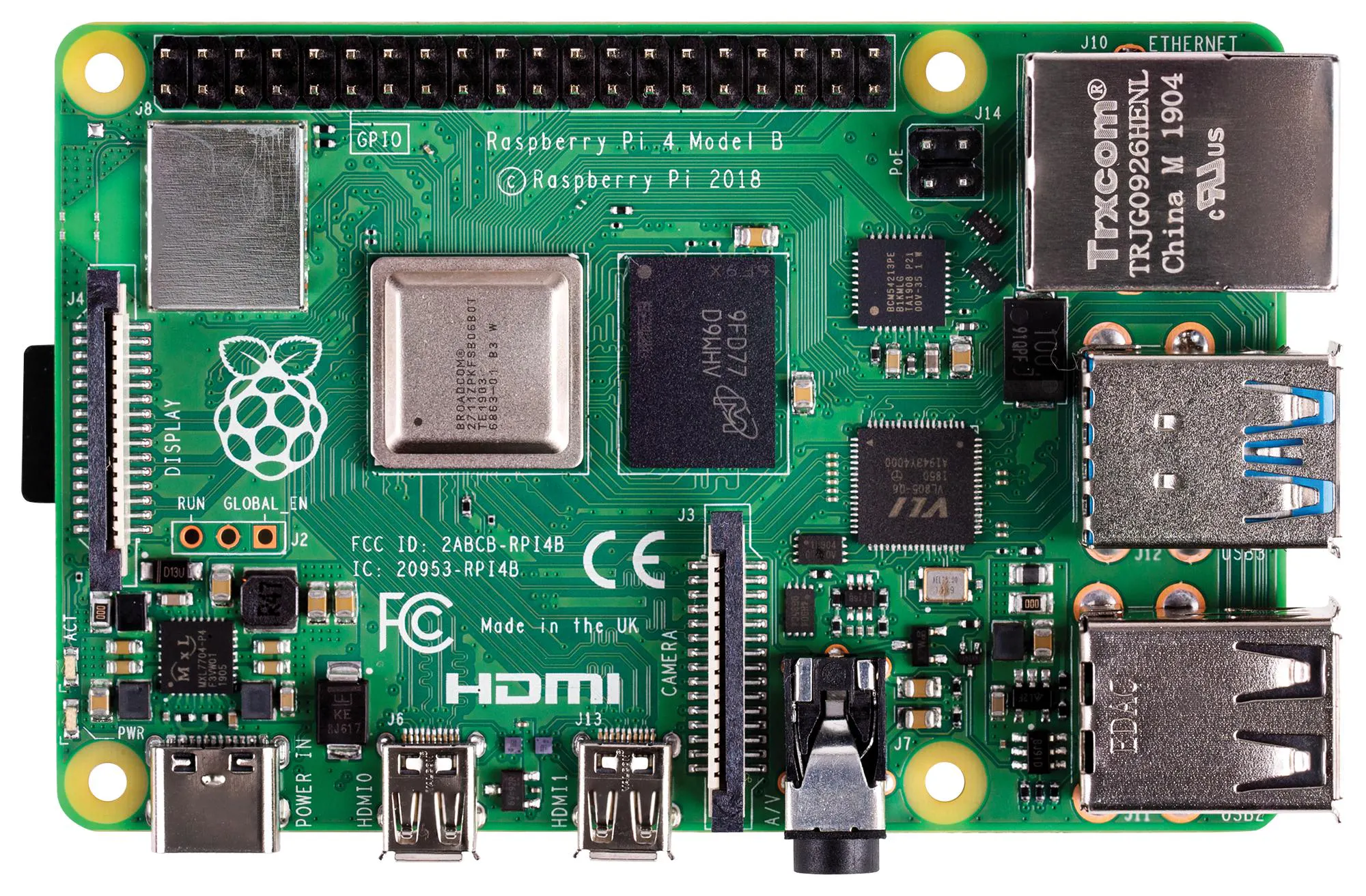# Thr33p10 – Third Hand Telepresence Bot

Building a smart little assistant to help with my videos!

IntermediateWork in progress3 hours1,753## Things used in this project

### Hardware componentsMATRIX Labs MATRIX Creator
×1Raspberry Pi 4 Model B
×1
 Servo Module (Generic)
×2
 Third hand (generic)
×1

## Custom parts and enclosures

### Servo wrist

Servo-to-third-hand joiner

### Ring holder

Screw-adjustable holder for MATRIX Creator (with third-hand attachment)

## Code

JavaScript
Run the servos with MATRIX Lite! Save this into your lite-js folder, then run "node servotest.js" and watch it go! (You can also substitute in other values, to test the limits of your servos.)
```const matrix = require("@matrix-io/matrix-lite");

matrix.gpio.setFunction(0, "PWM");
matrix.gpio.setFunction(1, "PWM");

matrix.gpio.setMode(0, "output");
matrix.gpio.setMode(1, "output");

matrix.gpio.setServoAngle({
pin: 0,
angle: 90,
min_pulse_ms: 0.8
});

matrix.gpio.setServoAngle({
pin: 1,
angle: 90,
min_pulse_ms: 0.8
});
```

### NFC-secured telepresence (MATRIX)

JavaScript
Launches the letsrobot.tv script, and shows rainbow lights, if the correct NFC token is presented.
```const matrix = require("@matrix-io/matrix-lite");
const shell = require('shelljs')
const nfc = require("@matrix-io/matrix-lite-nfc");

var mode = 0;
everLoop();

if (code === 256){
console.log("Tag Was Scanned");
console.log(tag);
console.log(tag.info.UID);
if (tag.info.UID === "LAUNCH-KEY"){
shell.exec('/home/pi/start_robot');
mode = 1;
}
if (tag.info.UID === "KILL-KEY"){
shell.exec('~/runmyrobot/scripts/kill_command');
mode = 0;
}
}

else if (code === 1024)
console.log("Nothing Was Scanned");
});

/* here beginneth the everloop function */

function everLoop() {
let everloop = new Array(matrix.led.length);

if (everloop.length == 35) {
ledAdjust = 0.51; // MATRIX Creator
} else {
ledAdjust = 1.01; // MATRIX Voice
}

let frequency = 0.375;
let counter = 0.0;
let tick = everloop.length - 1;

setInterval(()=>{
if (mode === 1){
// Create rainbow
for(i = 0; i < everloop.length; i++) {
let led = {};

led.r = Math.round(Math.max(0, (Math.sin(frequency*counter+(Math.PI/180*240))*155+100)/10));
led.g = Math.round(Math.max(0, (Math.sin(frequency*counter+(Math.PI/180*120))*155+100)/10));
led.b = Math.round(Math.max(0, (Math.sin(frequency*counter)*155+100)/10));

everloop[i] = led;
};

// Slowly show rainbow
if (tick != 0) {
for (i = tick; i > 0; i--) {
everloop[i] = {};
}
tick--;
}

matrix.led.set(everloop);

}
else {
matrix.led.set("black");
}

},200);
}
```

## Credits

### Alex Glow

98 projects • 978 followers
The Hackster team's resident Hardware Nerd. I like robots, music, EEG, wearables, and languages. FIRST Robotics kid.